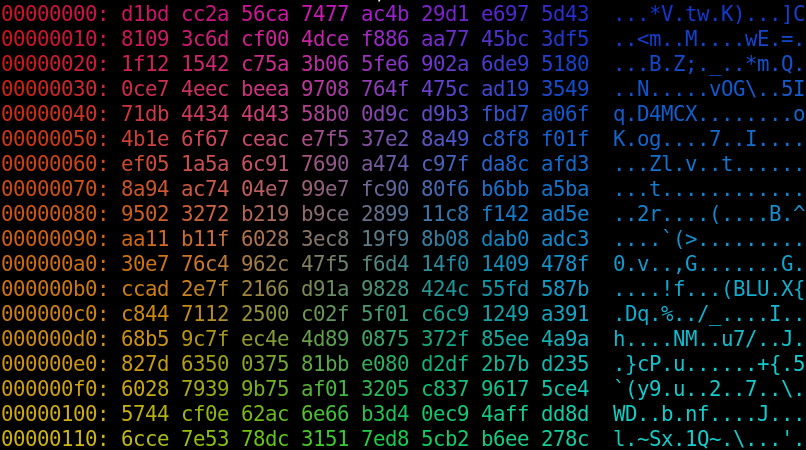# pretty rainbow colors with lolcat.sh

lolcat.sh is a tiny clone of lolcat that runs standalone on any POSIX-compatible system. the original program is written in ruby, which isn't on some computers i use, so i figured i would write something that works basically anywhere.

it is written mostly in standard awk and outputs fancy 24-bit terminal color codes.ascii art rainbows with default settings!

## usage

lolcat.sh

run the program with its files as standard input. any arguments to the command are sent to the inner code, which allows you to adjust display parameters. this also lets you run arbitrary code, so don't take them unfilitered from untrusted input. you also can't give just it a simple filename as an argument; pipe it directly with `./lolcat.sh < file` or similar.

``./lolcat.sh -v angle=60 -v angle_phase=0 -v width_phase=0 < file-to-display``

## one-line version

``awk -v angle=45 -v angle_phase=40 -v reverse=0 -v offset="" -v offset_phase=0 -v width=100 -v width_phase=8 "\$@" 'function put_rgb(color) { printf "\033[%s38;2;%d;%d;%dm", reverse ? "7;" : "", int(color * 255), int(color * 255), int(color * 255); } function hsv_to_rgb(hsv, rgb, c, h2, x) { c = hsv * hsv; h2 = hsv * 6; x = c * (1 - ((h2 % 2) - 1 > 0 ? (h2 % 2) - 1 : 1 - (h2 % 2))); if (int(h2) % 6 == 0) { rgb = c; rgb = x; rgb = 0; } else if (int(h2) % 6 == 1) { rgb = x; rgb = c; rgb = 0; } else if (int(h2) % 6 == 2) { rgb = 0; rgb = c; rgb = x; } else if (int(h2) % 6 == 3) { rgb = 0; rgb = x; rgb = c; } else if (int(h2) % 6 == 4) { rgb = x; rgb = 0; rgb = c; } else if (int(h2) % 6 == 5) { rgb = c; rgb = 0; rgb = x; } rgb += hsv - c; rgb += hsv - c; rgb += hsv - c; } BEGIN { srand(); if (offset == "") offset = rand() * 360; } { y = NR - 1; for (x = 0; x < length(\$0); x++) { for (i = 0; i < 3; i++) { mult_x = cos((angle + i * angle_phase) / 57.2976); mult_y = sin((angle + i * angle_phase) / 57.2976); hsv = (((x * mult_x + y * mult_y) / (width + width_phase * i)) % 1 + 1 + (offset + offset_phase * i) / 360) % 1; hsv = 0.8; hsv = 0.9; hsv_to_rgb(hsv, trgb); rgb[i + 1] = trgb[i + 1]; } put_rgb(rgb); printf("%s", substr(\$0, x + 1, 1)); } print "\033[0m"; }'``